Core java - Interview Questions and Answers for 'Dmx technologies' | Search Java Interview Question - javasearch.buggybread.com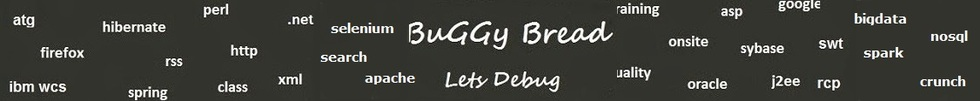# Search Interview QuestionsMore than 3000 questions in repository.There are more than 900 unanswered questions.Have a video suggestion.
Click Correct / Improve and please let us know.
Label / Company      Label / Company / TextSubmit Question

Core java - Interview Questions and Answers for 'Dmx technologies' - 2 question(s) found - Order By Newest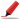Frequently asked in face to face interviews.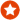Q1. Write a program to print fibonacci series.Core Java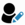Ans. int count = 15;
int[] fibonacci = new int[count];
fibonacci = 0;
fibonacci = 1;
for(int x=2; x < count; x++){
fibonacci[x] = fibonacci[x-1] + fibonacci[x-2];
}

for(int x=0; x< count; x++){
System.out.print(fibonacci[x] + " ");
}Help us improve. Please let us know the company, where you were asked this question :LikeDiscussCorrect / Improveebay   fibonacci series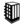Asked in 66 Companies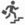basic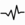frequentRelated Questions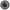What are the steps to be performed while coding Junit with Mocking framework ?What is a Sequence File?What different level of logging you use while coding ?Have you ever encoded the response before sending it back from the service? If Yes , Which encoding was used ?What are the different type of encoding you have used ?How does encoding affect using Reader / writer classes or Stream classes in Java ?What is the difference between html encoding and url encoding ?Does spaces get's encoded in html encoding ?Q2. Write a Program to print factorial of a number using recursionCore Java2017-01-29 17:47:20public static void main(String args[]) {
System.out.println(factorial(5));
}

private static int factorial(int number){
if(number == 1){
return 1;
} else {
return number * factorial(number - 1);
}
}
}Help us improve. Please let us know the company, where you were asked this question :LikeDiscussCorrect / ImproverecursionAsked in 24 CompaniesRelated QuestionsWhat will happen if we don't have termination statement in recursion ?Find Fibonacci first n numbers using recursion ?Write an Algorithm for Graph Traversal ? The Graph has a loop.Write a program to print sum of numbers between the start and end number

For example - Passing start number as 2 and end number as 10, it should print 2+3+4+5+6+7+8+9+10 = 54Write a program using Recursion to print multiplication of numbers between the start and end number

For example - Passing start number as 2 and end number as 10, it should print 2*3*4*5*6*7*8*9*10 = 3628800What is recursion in Java ?What will happen if we don't have termination statement in recursion ?Which of the two - iteration or recursion - is slower ?Write a Program to print fibonacci series using recursion.

## Help us and Others Improve. Please let us know the questions asked in any of your previous interview.

Any input from you will be highly appreciated and It will unlock the application for 10 more requests.